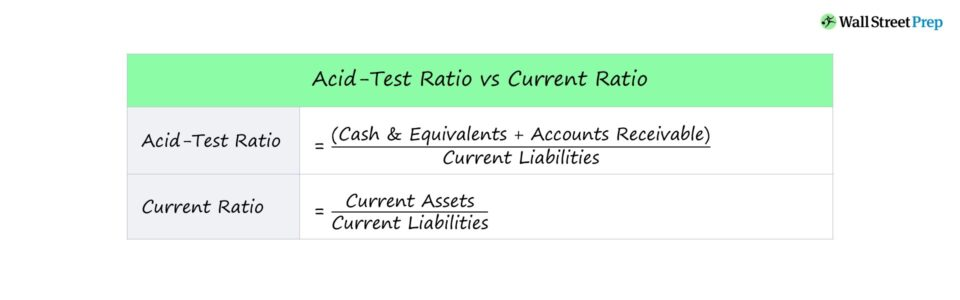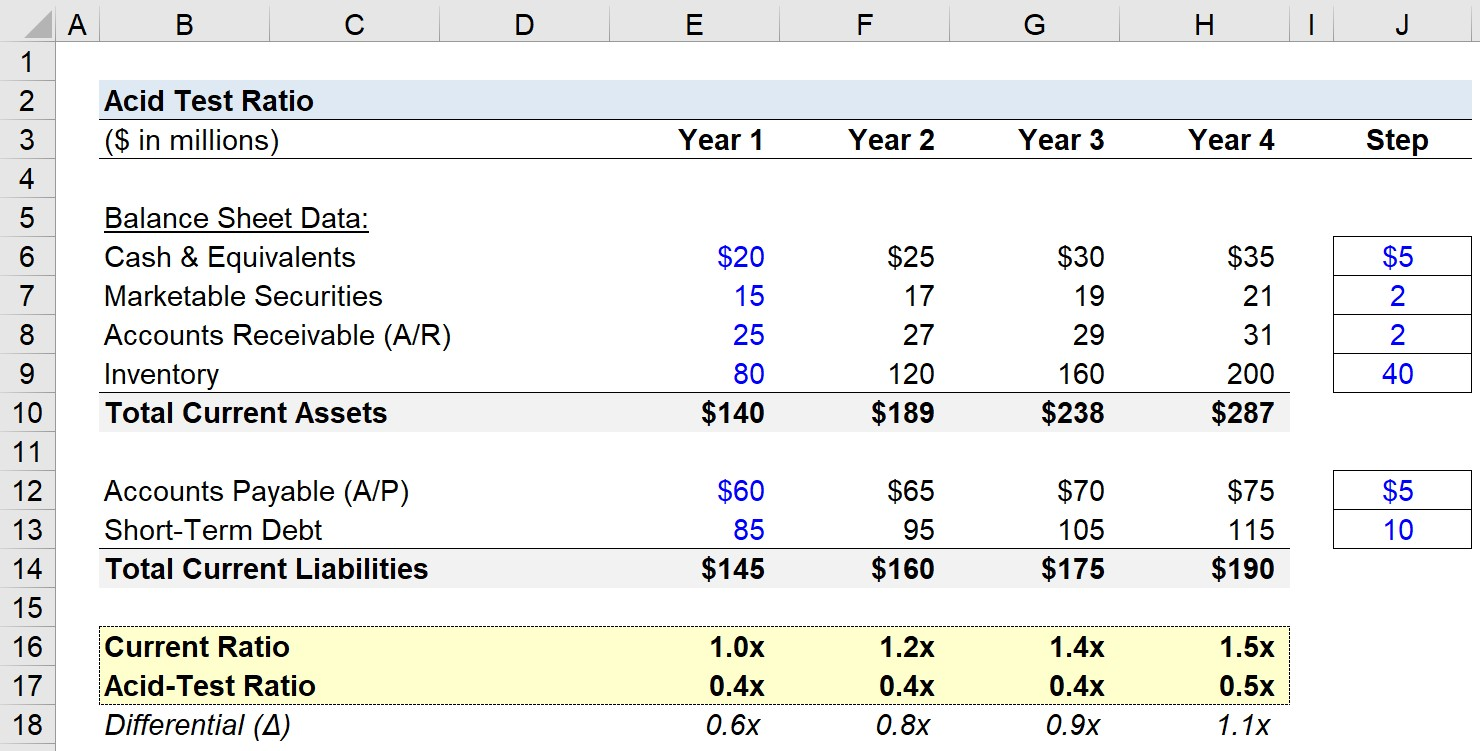# Acid Test Ratio

Guide to Understanding the Acid Test Ratio## How to Calculate Acid Test Ratio (Step-by-Step)

Liquidity is among one of the most important aspects of a company and its long-term viability.

The acid-test ratio and current ratio are two frequently used metrics to measure near-term liquidity risk, or a company’s ability to quickly pay off liabilities coming due in the next twelve months.

The steps to calculate the two metrics are similar, although the noteworthy difference is that illiquid current assets — e.g. inventory — are excluded in the acid-test ratio.

## Acid Test Ratio Formula

The formula for calculating the acid test (i.e. the quick ratio) and the current ratio for comparison is as follows.

Acid Test Ratio = Cash and Cash Equivalents + Accounts Receivable
Current Ratio = Current Assets ÷ Current Liabilities

As one would reasonably expect, the value of the acid-test ratio will be a lower figure since fewer assets are included in the numerator.

Hence, the acid-test ratio is more conservative in terms of what is classified as a current asset in the formula.

The acid-test ratio compares the near-term assets of a company to its short-term liabilities to assess if the company in question has sufficient cash to pay off its short-term liabilities.

Liquid Current Assets in Acid-Test Ratio:

Current Assets in Current Ratio:

• Cash & Equivalents
• Marketable Securities
• Accounts Receivable (A/R)
• Inventory
• Other Current Assets

If a company were to suddenly be required to pay off the entirety of its short-term liabilities, inventory is usually more time-consuming to liquidate (i.e. to sell and turn into cash) compared to marketable securities — which is where the acid-test ratio comes in.

## Liquidity Analysis: Acid Test Ratio Calculation Example

For example, imagine you’re tasked with calculating a company’s acid test ratio given the following balance sheet data:

Current Assets:

• Cash = \$20 million
• Marketable Securities = \$15 million
• Accounts Receivable (A/R) = \$25 million
• Inventory = \$60 million

Here, the total current assets are \$120 million and the liquid current assets is \$60 million.

Current Liabilities:

• Accounts Payables = \$30 million
• Short-Term Debt = \$25 million

Then, the required inputs are entered into the two formulas:

• Acid-Test Ratio = (\$20 million + \$15 million + \$25 million) ÷ (\$30 million + \$10 million) = 1.5x
• Current Ratio = (\$20 million + \$15 million + \$25 million + \$60 million) ÷ (\$30 million + \$10 million) = 3.0x

The current ratio in our example calculation is 3.0x while the acid-test ratio is 1.5x, which is attributable to the inclusion (or exclusion) of inventory in the respective calculations.

## How to Interpret Acid-Test Ratio (High or Low)

For both the acid-test ratio and current ratio, the same two general rules apply:

• Higher Ratio → Increased Coverage of Current Liabilities
• Lower Ratio → Decreased Coverage of Current Liabilities

Either liquidity ratio indicates whether a company — post-liquidation of its current assets — is going to have sufficient cash to pay off its near-term liabilities.

Therefore, the higher the ratio, the better the short-term liquidity health of the company.

The “floor” for both the quick ratio and current ratio is 1.0x, but this is the bare minimum, and higher values should be targeted.

In particular, a current ratio below 1.0x would be more concerning than a quick ratio below 1.0x, although either ratio being low could be a sign that liquidity might soon become a concern.

A company with a low current or quick ratio should likely proceed with some degree of caution, and the next step would be to determine how much more capital and how quickly it could be obtained.

## Acid Test Ratio Calculator — Excel Template

We’ll now move to a modeling exercise, which you can access by filling out the form below.Submitting ...

## Acid Test Ratio Calculation Example

The model assumptions we’ll be using for our hypothetical company can be found below.

Current Assets:

• Cash and Cash Equivalents: \$20m — Increases by \$5m / Year
• Marketable Securities: \$15m — Increases by \$2m / Year
• Accounts Receivable (A/R): \$25m — Increases by \$2m / Year
• Inventory: \$80m — Increases by \$40m / Year

Current Liabilities:

• Accounts Payable: \$65m — Increases by \$5m / Year
• Short-Term Debt: \$85m — Increases by \$10m / Year

In Year 1, the current ratio can be calculated by dividing the sum of the liquid assets by the current liabilities.

• Current Ratio — Year 1: (\$20m Cash + \$15m Marketable Securities + \$25m A/R + \$80m Inventory) ÷ \$145m (Current Liabilities) = 1.0x

Next, we apply the acid-test ratio formula in the same time period, which excludes inventory, as mentioned earlier.

• Quick Ratio — Year 1: (\$20m Cash + \$15m Marketable Securities + \$25m A/R) ÷ \$145m (Current Liabilities) = 0.4x

From Year 1 to Year 4, the following changes occur across the forecast period:

• Current Ratio: 1.0x → 1.5x
• Acid-Test Ratio: 0.4x → 0.5x

The current ratio is benefiting from the growth in the inventory balance, which is beneficial for debt lenders requiring capital (i.e. collateral), but the accumulation of inventory usually results from lower revenue due to lower market demand.

Since the target range for the current ratio is between 1.5x to 3.0x, the company appears to be in relatively good health based on its current ratio.

However, the acid-test ratio implies a different story regarding the liquidity of the company, as it is below 1.0x.

In closing, we can see the potentially significant differences that may arise between the two liquidity ratios due to the inclusion or exclusion of inventory in the calculation of current assets.Step-by-Step Online Course

### Everything You Need To Master Financial Modeling

Enroll in The Premium Package: Learn Financial Statement Modeling, DCF, M&A, LBO and Comps. The same training program used at top investment banks.Inline FeedbacksMay 19, 2023 11:48 pm

Very interesting explanations, easy to understand from basic to advance .Keep it up.May 22, 2023 3:33 pm

Thanks, JohnsonFolafoluwa
November 19, 2022 1:58 am

I’m so gratefulNovember 21, 2022 3:19 pmLearn Financial Modeling Online

Everything you need to master financial and valuation modeling: 3-Statement Modeling, DCF, Comps, M&A and LBO.

X

The Wall Street Prep Quicklesson Series

7 Free Financial Modeling Lessons

Get instant access to video lessons taught by experienced investment bankers. Learn financial statement modeling, DCF, M&A, LBO, Comps and Excel shortcuts.